Building a winning crypto portfolio. Part 1: Modern Portfolio Theory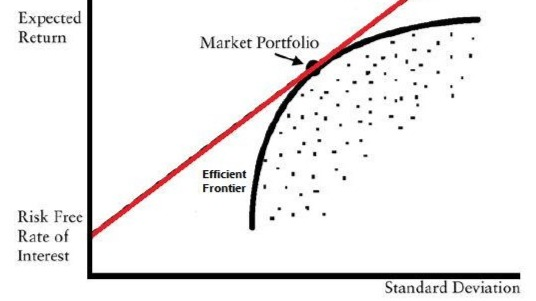Building a winning crypto portfolio. Part 1: Modern Portfolio Theory

By Adamq | Investing Principles | 4 Apr 2020

In my previous article, I discussed an approach to investing that could allow you to remain calm through the COVID market meltdown: https://www.publish0x.com/investing-principles/guide-to-surviving-the-covid-19-market-meltdown-madness-xoeyqz

One of the strategies I discussed, was having a clear approach to constructing your portfolio. In this article I would like to double-click on this point. Firstly, in this article we will look at the theory behind this and then in my next article, we will look a little closer at the issues that we face in practise and particularly in applying it to crypto portfolios.

Modern Portfolio Theory

Modern portfolio theory or MPT is built on a few core concepts. In words, it is about maximising the return that can be achieved per unit of risk taken in a portfolio. The objective is to maximise this outcome, given the investor’s risk tolerance. Clearly a portfolio suitable for widows and orphans (who have lower risk tolerance) will look completely different to that for a high flying Silicon Valley executive (who presumably has a higher risk tolerance). Such a portfolio which maximises return per unit of risk taken is known as an efficient portfolio.

So how do we measure risk? MPT assumes that returns achieved by a security follow a lognormal distribution. It’s a technical point but for your understanding just assume that returns are normally distributed (lognormal is used since the price of a security can’t fall below zero). Risk is defined as the standard deviation (SD) of this distribution. The standard distribution measures the dispersion of values around the central value or mean (average). If the distribution is wider, then returns can be further from the mean and SD is larger – this security has higher risk. If the distribution is narrower, then the returns are closer to the mean and SD is smaller – this security has lower risk.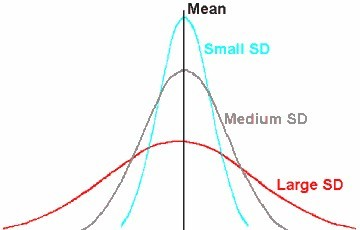Fig 1. Measuring the riskiness of an asset

If a portfolio is constructed from two securities, KA and KB, with weightings of WA and WB and correlation between them of R(KA,KB ), then the standard deviation (or risk) of the combined portfolio, σP, is given by the equation: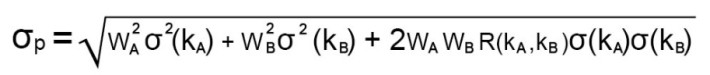Fig 2. Riskiness of a portfolio

Now before your eyes glaze over and you run to the hills to hide, let me explain what is going on here through some examples.

Example 1 - Two securities that are independent of each other

Ok so what does it mean if two securities are independent? It means that their returns are uncorrelated. Correlation measures the degree to which the returns of the securities move together (i.e. if the return on the first security is high then the return on the second security will also be high). This happens when the securities have similar drivers. For example, I might expect the returns for an airline to have some correlation with that of a utility. Why? Well when the oil price goes up, we expect the operating costs for an airline to also go up and for stock to perform less well. Similarly, if a utility is burning oil or gas to make electricity, we would also expect its costs to go up with the oil price and for its stock to perform less well. In this instance, we would expect the stocks to have a positive correlation.

In our first example we assume that there is no correlation between the stocks and so the term containing R(KA,KB ) becomes zero and the formula simplifies to just the first two terms. Here the risk is given by the square root of the weighted sum of the square of the risks of each security. So why do I need to square the risks? Well this is due a property of the normal distribution, where the returns can be either side of the mean (some will be positive relative to it and some will be negative relative to it) and we need a measure that just looks at the average distance (i.e. dispersion) from the mean rather than whether it higher than or lower than the mean. When I square a negative number, I get a positive number and then the square root is positive. This is the same result as squaring a positive number of the same value and then taking the square root.

Ok, so what happens if I invest half my money in each of two stocks that are independent. Here I am taking Microsoft and P&G as examples of two potentially uncorrelated stocks. Note that I have made up the numbers for the sake of this example.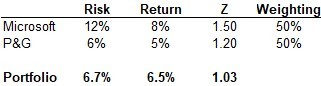Table 1. Portfolio of independent or uncorrelated assets

First thing we see is that return on owning the two stocks is simply the average return (no surprise here), but interestingly the portfolio risk is closer to that of the less risky stock. Is this delivering me any benefits? Well it becomes more interesting when we look at the return we are getting for each unit of risk taken. The measure of this is Z, which is the risk divided by the return and is known as the Sharpe Ratio of the asset or portfolio. Here we note that Z for the portfolio is lower than that of either of the two individual stocks. This means that we are taking less risk per unit of return achieved and therefore owning the two stocks is more efficient than owning either stocks individually.

This property is an illustration of the diversification benefit achieved from owning multiple stocks in a portfolio. As we add more and more stocks to the portfolio, we can get increased benefit from diversification, up to a natural limit known as the market risk of the portfolio. At this point, we have diversified away all unsystematic (or stock specific) risks and we are just left with the underlying risk inherent in the market. This can be achieved by constructing a portfolio with a large number of stocks. Rule of thumb here is that ~10 stocks is usually sufficient to achieve this benefit

Example 2 - Two positively correlated stocks

As a second example let’s consider two stocks that are positively correlated. Remember this means that they are subject to the same drivers and therefore they will tend to rise and fall together.

In this case, lets take hypothetical risk and returns for Google and Microsoft. We expect these stocks as tech stocks to be somewhat correlated to each other, since they are likely to have the same underlying performance drivers. To get a baseline, lets firstly assume that they are not correlated (i.e. that they are independent):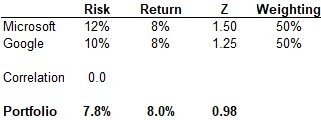Table 2a. Baseline for positively correlated assets

Here, as with example 1, we see that the Sharpe Ratio for the portfolio is lower than for the stocks individually and so we are getting some benefits from diversification. Now let’s assume that there is a correlation of 0.7 between the stocks, which means that 70% of the rises and falls of one of the stocks, will match the rise and falls of the other stock: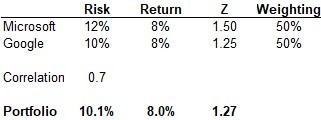Table 2b. Portfolio of positively correlated assets

In this case, we see that portfolio has a higher Sharpe Ratio than if they were uncorrelated (our baseline). On the surface, it might not seem like a good idea to own this portfolio, since it would be more efficient to just own the Google stock on its own. However, imagine that I was an employee of Microsoft and received stock options. In this case, I could achieve a better risk return trade-off by purchasing a similar amount of Google stock and so I might still wish to hold this portfolio. If I already had stock in Google the same would not be the case as I would worsen my risk-return trade-off since Z would be higher.

Typically, we want to avoid having stocks in a portfolio that are all positive correlated, since we worsen our trade-off between risk and return. This is not typically the way we can construct an efficient portfolio.

Example 3 – Two negatively correlated stocks

In this example lets consider an airline and a gold mining company. Again, I have made up the risk and return figures to illustrate the point. As we previously noted, when oil prices rise, we would expect the stock performance of an airline to fall. However, when oil prices rise, the cost of goods and services across the economy also rises (since the costs for a trucking company that is delivering consumer goods and food to the grocery also increases and the costs of manufacturing those goods would also increase). This increase in the general price of things is known as inflation. Gold is generally seen as a hedge against inflation since theoretically its intrinsic value remains the same (i.e. an ounce of gold is simply worth what an ounce of gold is worth). So, in the case of inflation, where the purchasing power of my money has declined, the price of purchasing gold (in my less valuable dollars) goes up. In this way we can see that as oil prices go up, we can expect the return from gold and miners producing gold to also go up. So, we would expect the stock performance of the airline (lets say AA) and the gold producer (lets say Newmont) to be negatively correlated.

Lets first look at the case where we assume no correlation, so we have a baseline for performance: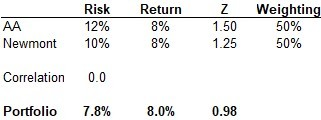Table 3a. Baseline for negatively correlated assets

Again, we see that it is more efficient to hold the two stocks than it is to hold either alone. But look at what happens when we assume a negative correlation.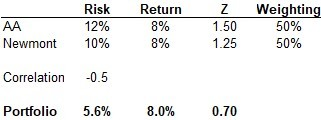Table 3b. Portfolio of negatively correlated assets

Here we see that the risk return trade-off for the portfolio has significantly improved and this is a much more efficient portfolio.

Conclusion

MPT shows that we can gain diversification benefits from holding multiple uncorrelated stocks. In the real world, many stocks are correlated, since their underlying performance is linked to common drivers. If the correlation is positive, we lose some of the benefits of diversification and can in some instance achieve a worse outcome than holding a more concentrated portfolio. However, the holy grail of portfolio construction is to find stocks or different asset types that are negatively correlated. When we can construct portfolios with negatively correlated assets, the diversification benefits we can achieve are greatly magnified and the efficiency of our portfolio improved. This is because the assets hedge one another. When one performs badly, the other performs well and so the overall portfolio performance is improved.

In my next article, I will discuss some of the issues that occur with MPT and look to how we can apply this theory to constructing a crypto portfolio. Stay tuned!Strategy consultant, cryptoenthusiast and amateur author.Investing Principles

In this blog, I aim to share my views on how to successfully invest in crypto for the long-term.Send a \$0.01 microtip in crypto to the author, and earn yourself as you read!

20% to author / 80% to me.
We pay the tips from our rewards pool.